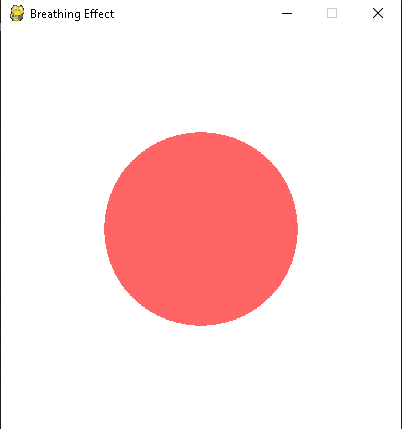# 如何使用Pygame添加颜色呼吸效果？

Pygame是一款流行的Python游戏开发库，提供了广泛的函数用于创建2D游戏。它使用低级多媒体库SDL来处理游戏中的图形、声音和输入。Pygame库能够轻松直观地创建基于Python的游戏。

## 创建呼吸效果

### 将呼吸效果应用于游戏对象

• 导入必要的库（Pygame、math）。
• 初始化Pygame。
• 设置游戏屏幕。
• 定义游戏对象。
• 设置初始颜色和缩放值。
• 进入游戏循环：
• 处理事件，包括关闭按钮事件。
• 更新颜色和缩放值。
• 清空屏幕。
• 绘制呼吸对象。
• 更新屏幕。
• 控制帧率。
• 关闭游戏时退出Pygame。

### 示例

import pygame
import math

# Initialize Pygame
pygame.init()

# Set up the screen
screen = pygame.display.set_mode((400, 400))
pygame.display.set_caption("Breathing Effect")


# Set up colors
color = pygame.Color(255, 0, 0)  # Starting color
max_scale = 2.0  # Maximum scaling factor
min_scale = 1.0  # Minimum scaling factor
scale_step = 0.01  # Scaling factor change per frame
max_alpha = 255  # Maximum alpha value for color
min_alpha = 50   # Minimum alpha value for color
alpha_step = 1   # Alpha value change per frame


# Set up clock
clock = pygame.time.Clock()

# Main game loop
while True:
for event in pygame.event.get():
if event.type == pygame.QUIT:
pygame.quit()
sys.exit()


# Update the scale factor of the circle
scale = min_scale + (max_scale - min_scale) * 0.5 * (1 + math.sin(pygame.time.get_ticks() * scale_step))

# Update color's alpha value
alpha = min_alpha + (max_alpha - min_alpha) * 0.5 * (1 + math.sin(pygame.time.get_ticks() * alpha_step))
color.a = int(alpha)  # Convert alpha value to integer

# Clear the screen
screen.fill((255, 255, 255))


# Draw a circle with the changing scale and color
circle_size = int(50 * scale)
circle_surface = pygame.Surface((circle_size * 2, circle_size * 2), pygame.SRCALPHA)
pygame.draw.circle(circle_surface, color, (circle_size, circle_size), circle_size)
screen.blit(circle_surface, (200 - circle_size, 200 - circle_size))

# Update the screen
pygame.display.flip()

# Control the frame rate
clock.tick(30)  # Adjust this value to control the speed of the breathing effect


### 输出• 回顶